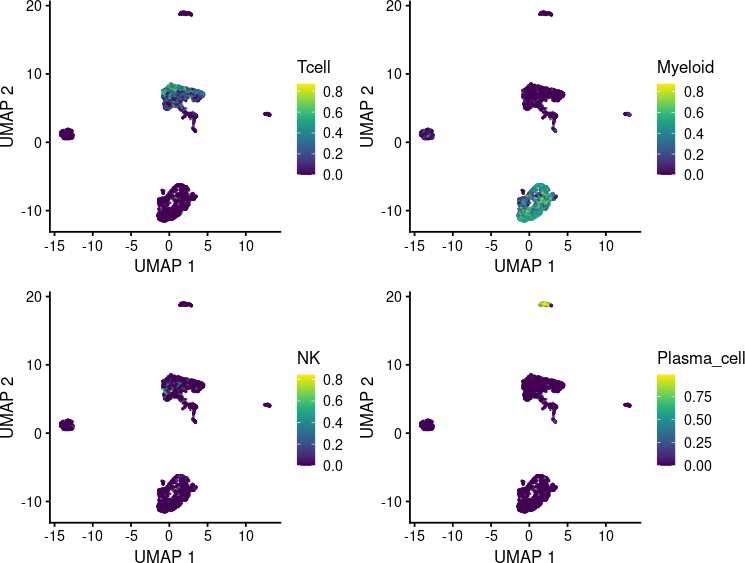# 1 Introduction

SingleCellExperiment is Bioconductor’s data structure of choice for storing single-cell experiment data. The function `ScoreSignatures_UCell()` allows performing signature scoring with UCell directly on `sce` objects. UCell scores are returned in a altExp object: `altExp(sce, 'UCell')`

# 2 Get some testing data

For this demo, we will download a single-cell dataset of lung cancer (Zilionis et al. (2019) Immunity) through the scRNA-seq package. This dataset contains >170,000 single cells; for the sake of simplicity, in this demo will we focus on immune cells, according to the annotations by the authors, and downsample to 5000 cells.

``````library(scRNAseq)
lung <- ZilionisLungData()
immune <- lung\$Used & lung\$used_in_NSCLC_immune
lung <- lung[,immune]
lung <- lung[,1:5000]

exp.mat <- Matrix::Matrix(counts(lung),sparse = TRUE)
colnames(exp.mat) <- paste0(colnames(exp.mat), seq(1,ncol(exp.mat)))``````

# 3 Define gene signatures

Here we define some simple gene sets based on the “Human Cell Landscape” signatures Han et al. (2020) Nature. You may edit existing signatures, or add new one as elements in a list.

``````signatures <- list(
Tcell = c("CD3D","CD3E","CD3G","CD2","TRAC"),
Myeloid = c("CD14","LYZ","CSF1R","FCER1G","SPI1","LCK-"),
NK = c("KLRD1","NCR1","NKG7","CD3D-","CD3E-"),
Plasma_cell = c("MZB1","DERL3","CD19-")
)``````

# 4 Run UCell on sce object

``````library(UCell)
library(SingleCellExperiment)

sce <- SingleCellExperiment(list(counts=exp.mat))
sce <- ScoreSignatures_UCell(sce, features=signatures,
assay = 'counts', name=NULL)
altExp(sce, 'UCell')``````
``````## class: SummarizedExperiment
## dim: 4 5000
## assays(1): UCell
## rownames(4): Tcell Myeloid NK Plasma_cell
## rowData names(0):
## colnames(5000): bcHTNA1 bcHNVA2 ... bcGVZB4999 bcHGKL5000
## colData names(0):``````

Dimensionality reduction and visualization

``````library(scater)
library(patchwork)
#PCA
sce <- logNormCounts(sce)
sce <- runPCA(sce, scale=TRUE, ncomponents=10)

#UMAP
set.seed(1234)
sce <- runUMAP(sce, dimred="PCA")``````

Visualize UCell scores on low-dimensional representation (UMAP)

``````pll <- lapply(names(signatures), function(x) {
plotUMAP(sce, colour_by = x, by_exprs_values = "UCell",
point_size=0.2) + theme(aspect.ratio = 1)
})
wrap_plots(pll)``````# 5 Signature smoothing

Single-cell data are sparse. It can be useful to ‘impute’ scores by neighboring cells and partially correct this sparsity. The function `SmoothKNN` performs smoothing of single-cell scores by weighted average of the k-nearest neighbors in a given dimensionality reduction. It can be applied directly on SingleCellExperiment objects to smooth UCell scores:

``sce <- SmoothKNN(sce, signature.names = names(signatures), reduction="PCA")``
``````a <- plotUMAP(sce, colour_by="Myeloid", by_exprs_values="UCell",
point_size=0.2) + ggtitle("UCell") + theme(aspect.ratio = 1)

b <- plotUMAP(sce, colour_by="Myeloid_kNN", by_exprs_values="UCell_kNN",
point_size=0.2) + ggtitle("Smoothed UCell") + theme(aspect.ratio = 1)

a | b``````